# monocone

Create monocone antenna on circular ground plane

## Description

The `monocone` object creates a monocone antenna on a circular ground plane. A classical monocone antenna consists of a cone and a ground plane. To increase the bandwidth of the antenna, you can modify the antenna by merging the cone with a circular cylinder. By default, the `monocone` object creates the modified version.Create a classical monocone antenna (without the cylinder on top) using one of these methods:

• Set the height of the antenna to equal the sum of the cone height and the feed height.

• Set the cone height to equal half of the difference between the total height and the feed height. Then set the radius at the aperture to twice the radius at the junction.

## Creation

### Syntax

``ant = monocone``
``ant = monocone(Name,Value)``

### Description

example

````ant = monocone` creates a monocone antenna with the feedpoint at the center of the ground plane. The default dimensions are for a resonant frequency of 3.8 GHz.```

example

````ant = monocone(Name,Value)` sets properties using one or more name-value pairs. For example, ```ant = monocone('Height',0.0560)``` creates a monocone antenna with a total height of 0.0560 meters.```

## Properties

expand all

Antenna radii, specified as a three-element real vector with each element unit in meters.

• The first element represents the narrow radius of the cone.

• The second element represents the radius at the junction of the cone and the cylinder.

• The third element represents the radius at the top of the cylinder.

Example: ```'Radii',[6.3300e-04 0.0546 0.0220]```

Example: ```ant.Radii = [6.3300e-04 0.0546 0.0220]```

Data Types: `double`

Total height of the antenna from the ground plane to the aperture of the antenna, specified as a positive scalar in meters.

Example: `'Height',0.0560`

Example: `ant.Height = 0.0560`

Data Types: `double`

Vertical height of the cone from the apex of the cone to the junction of the cone and the cylinder, specified as a positive scalar in meters.

Example: `'ConeHeight',0.02250`

Example: `ant.ConeHeight = 0.02250`

Data Types: `double`

Gap between the cone and the ground plane, specified as a positive scalar in meters.

Example: `'FeedHeight',0.0034`

Example: `ant.FeedHeight = 0.0034`

Data Types: `double`

Width of the feed, specified as a positive scalar in meters.

Example: `'FeedWidth',0.0050`

Example: `ant.FeedWidth = 0.0050`

Data Types: `double`

Radius of the ground plane, specified as a positive scalar in meters.

Example: `'GroundPlaneRadius',0.0050`

Example: `ant.GroundPlaneRadius = 0.050`

Data Types: `double`

Type of the metal used as a conductor, specified as a metal material object. You can choose any metal from the `MetalCatalog` or specify a metal of your choice. For more information, see `metal`. For more information on metal conductor meshing, see Meshing.

Example: ```m = metal('Copper'); 'Conductor',m```

Example: ```m = metal('Copper'); ant.Conductor = m```

Lumped elements added to the antenna feed, specified as a `lumpedElement` object. You can add a load anywhere on the surface of the antenna. By default, the load is at the feed. For more information, see `lumpedElement`.

Example: `'Load',lumpedElement`, where `lumpedElement` is load added to the antenna feed.

Example: `ant.Load = lumpedElement('Impedance',75)`

Tilt angle of the antenna, specified as a scalar or vector with each element unit in degrees. For more information, see Rotate Antennas and Arrays.

Example: `'Tilt',90`

Example: `ant.Tilt = 90`

Example: `'Tilt',[90 90]`,`'TiltAxis',[0 1 0;0 1 1]` tilts the antenna at 90 degrees about the two axes defined by the vectors.

Note

The `wireStack` antenna object only accepts the dot method to change its properties.

Data Types: `double`

Tilt axis of the antenna, specified as:

• Three-element vector of Cartesian coordinates in meters. In this case, each coordinate in the vector starts at the origin and lies along the specified points on the X-, Y-, and Z-axes.

• Two points in space, each specified as three-element vectors of Cartesian coordinates. In this case, the antenna rotates around the line joining the two points in space.

• A string input describing simple rotations around one of the principal axes, 'X', 'Y', or 'Z'.

Example: `'TiltAxis',[0 1 0]`

Example: `'TiltAxis',[0 0 0;0 1 0]`

Example: `ant.TiltAxis = 'Z'`

Note

The `wireStack` antenna object only accepts the dot method to change its properties.

Data Types: `double`

## Object Functions

 `coneangle2size` Calculates equivalent cone height, broad radius, and narrow radius for cone `show` Display antenna or array structure; display shape as filled patch `axialRatio` Axial ratio of antenna `beamwidth` Beamwidth of antenna `charge` Charge distribution on metal or dielectric antenna or array surface `current` Current distribution on metal or dielectric antenna or array surface `design` Design prototype antenna or arrays for resonance around specified frequency `efficiency` Radiation efficiency of antenna `EHfields` Electric and magnetic fields of antennas; Embedded electric and magnetic fields of antenna element in arrays `impedance` Input impedance of antenna; scan impedance of array `mesh` Mesh properties of metal or dielectric antenna or array structure `meshconfig` Change mesh mode of antenna structure `optimize` Optimize antenna or array using SADEA optimizer `pattern` Radiation pattern and phase of antenna or array; Embedded pattern of antenna element in array `patternAzimuth` Azimuth pattern of antenna or array `patternElevation` Elevation pattern of antenna or array `rcs` Calculate and plot radar cross section (RCS) of platform, antenna, or array `returnLoss` Return loss of antenna; scan return loss of array `sparameters` Calculate S-parameter for antenna and antenna array objects `vswr` Voltage standing wave ratio of antenna

## Examples

collapse all

Create and view a default monocone antenna.

`ant = monocone`
```ant = monocone with properties: Radii: [5.0000e-04 0.0110 0.0110] GroundPlaneRadius: 0.0325 ConeHeight: 0.0115 Height: 0.0250 FeedHeight: 5.0000e-04 FeedWidth: 5.0000e-04 Conductor: [1x1 metal] Tilt: 0 TiltAxis: [1 0 0] Load: [1x1 lumpedElement] ```
`show(ant)`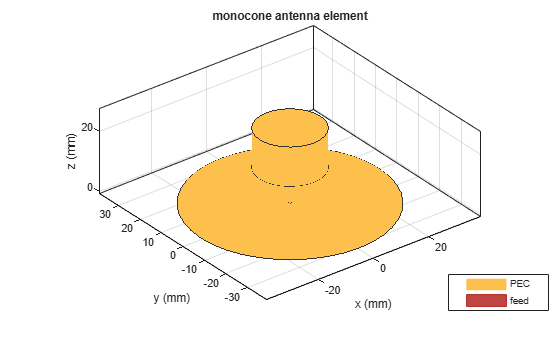Create a monocone antenna with an infinite ground plane.

```ant = monocone; ant.GroundPlaneRadius = inf; show(ant)```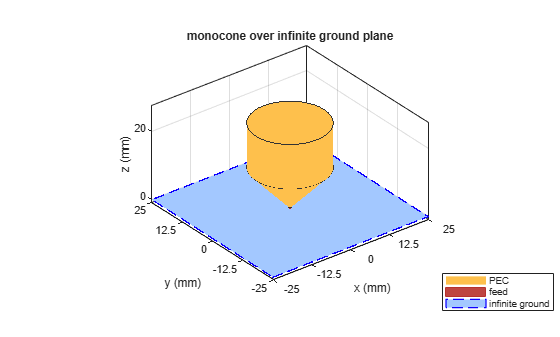Plot the radiation pattern of the monocone antenna for the given frequency.

`pattern(ant,3.94e9)`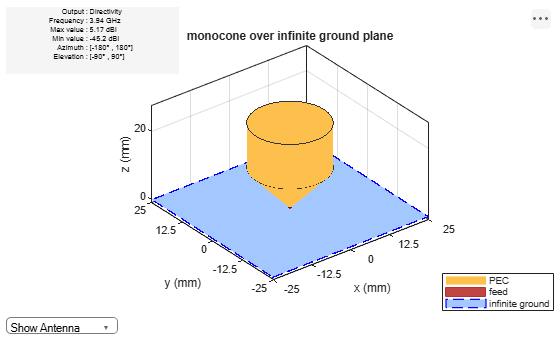Create a classical monocone antenna by setting the total height of the antenna to equal the sum of cone height and feed height.

```ant = monocone; ant.Height = ant.ConeHeight+ant.FeedHeight; show(ant)```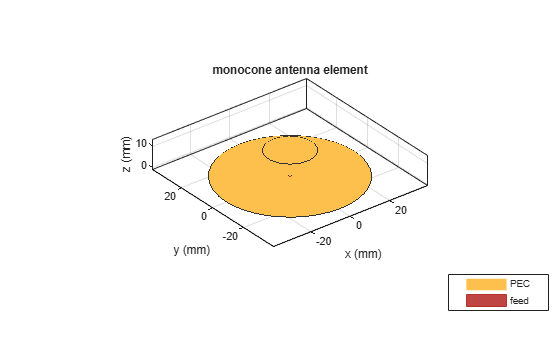Calculate antenna impedance over the given frequency span.

`impedance(ant,(1e9:0.1e9:6e9)) `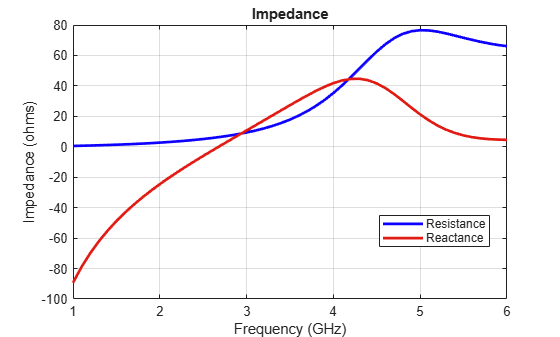McDonald, James L., and Dejan S. Filipovic. “On the Bandwidth of Monocone Antennas.” IEEE Transactions on Antennas and Propagation 56, no. 4 (April 2008): 1196–1201. https://doi.org/10.1109/TAP.2008.919226.